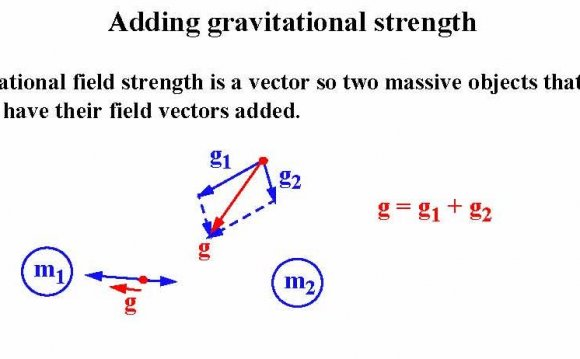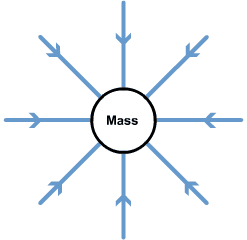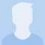# What is gravitational field strength?

January 31, 2023Gravity is the force that acts between any two masses, which means that you are actually attracted to all your friends, no matter how much you deny it!

Fortunately we are usually strong enough to overcome the attraction because it only becomes strong enough for us to notice its effects when the masses involved become very large e.g. planets or stars.

The force always attracts and never repels and it increases the nearer the two bodies are or the larger the masses of the bodies.The arrows show the direction of a force on a mass placed in the field. Note: the field is not uniform (the field lines are never parallel or equi-spaced) so as you move away from the object, the strength of the field reduces.

A definition for gravitational fields is: a region in which objects with mass experience a force.

The strength of a gravitational field is defined as the force, F, acting on a unit mass, m, in the field which in an equation is:

Symbol: g. Units: newtons per kilogram, Nkg-1, which is the same as ms-2.

Uniform field.

In a uniform field, g will remain constant. You can consider the Earth's gravitational field to be uniform as long as you don't move more than a few kilometres above the surface of the Earth. On the surface of the Earth, g = 9.81Nkg-1, of course.

It is clear looking at the diagram of field lines in the section above that the field varies as you move away from the object at its centre. In a radial field, the field strength reduces as you move away from the centre.

Newton was the first person to fully explain gravitational fields. He came up with the following equation for field strength in a radial field:

where.

g = field strength at a point

G = the universal gravitational constant, value 6.7 x 10-11 Nkg-2m2

m = the mass of the object which causes the field

r = the separation between that point and the centre if the object causing the field.

Note:.

g ∝ mass. So increase the mass and you increase the strength of the gravitational field.

. So increase the distance from the mass at the centre of the field and the field strength reduces. This is called an inverse square law as field strength is proportional to the inverse of the square of the separation of the masses. (For example if you go twice as far from a mass, its gravitational field is ¼ as strong).

Note: r is taken from the centre of gravity of the object, the point at which all the mass seems to be.

Source: www.s-cool.co.uk
##### INTERESTING VIDEORelationship between gravitational field strength and ...Gravitational Field Strength and Orbital MotionGravitational Field Strength

##### INTERESTING FACTS##### What is Pluto gravitational field strength?

Pluto's gravitational field strength is about 6.7% of the Earth's.